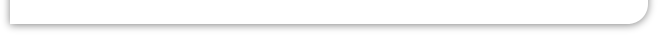Conditional Counting - COUNTIF Excel's COUNTIF function is often useful when analysing your data - To find the number of samples above or below a certain point, within a boundary, or for example negative or positive. Count Negative Readings: Here are a couple of examples: they both are being applied to a column of data in cells B2 to B100. To count the number of negative readings =COUNTIF(B2:B100,"<0") Count Number Of readings in a range: To count the number of readings which are between 17 and 18: =COUNTIF(B2:B100,">=17")-COUNTIF(B2:B100,"<18") Using the Functions in Macros: You can use COUNTIF in your VBA code.  Here we are counting the number of negative readings and storing the result in a variable called BelowFreezing. BelowFreezing = Application.COUNTIF(Sheets("Sheet1").Range("B2:B100"), "<0") Counting over Scattered Cells The COUNTIF function checks to see if the specified cells meet one condition.  You can use cell references as in our example, or a named range. The range of cells can spread several rows and columns, but must be contiguous: =COUNTIF(A2,A4,A6,"<0") will not work. However, you can sum COUNTIF functions to get the required result. =COUNTIF(A2,"<0")+COUNTIF(A4,"<0")+COUNTIF(A6,"<0") Having a Cell Contain the Condition: In the examples shown the conditions are entered as numbers. Instead a cell reference could be used. In this example the cell C1 holds the numerical part of the condition (the operand). Note the less than symbol is enclosed in inverted commas and the & sign which precedes the cell reference. =COUNTIF(A2:A100,"<"&C1) Counting against more than one Condition: With COUNTIF you cannot count against more than one condition: such as the number of times both the temperature was over 0 oC and the humidity was below 50%.  Instead you could use DCOUNT